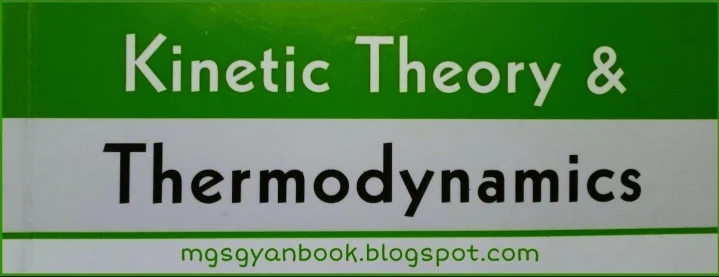# Physics 2nd,Unit-I (Kinetic Theory & Thermodynamics) For B.Sc. 1st. Year Students For All Affiliated College to M.G.K.V.P. University VaranasiThe gas which strictly obeys the gas laws (Boyle's laws, Charle's law etc.),at all tempratures and pressures, remaining as a gas, is known as perfect or ideal gas. The molecules of an ideal gas do not exert force of attraction on one another. Therefore, its molecules have only kinetic energy which depends on temperature. The gas equation for 1 mole of an ideal gas is,
pV = RT

where all symbols have their usual meanings.
All real gases deviate from these gas laws to a smaller or greater extent. The general nature of the deviations is small at low pressure and high temperature. but large at high pressure and low temperatures
. Also they can be liquified (even solidified) under appropriate conditions of temperature and pressure.

## Kinetic Theory of Matter

There is a close relationship between heat and mechanical energy. The nature of this relationship is given by the kinetic theory of matter. Because its explains the nature of heat, so may be called as kinetic theory of heat. It is based on following two postulates ;
1. Matter is not continuous but consist of very small particles in large number, called molecules. Even if molecules are in contact with each other there are interspaces in between them. A molecule is the smallest part of the substance having all of its characteristic properties and can have an independent existence.
2. The molecules are in a state of continuous motion. i.e., they move haphazardly with great speeds in all directions. the freedom of movement of the molecules is different in there states of matter. i.e.,solid,liquid and gas.
On the basis of kinetic theory, three states of matter are explained in the following way:

### 1. Solids

In solids, molecules are densely packed and exert a large cohesive force on each other. In this way molecules are nearly fixed in there position and can only oscillate about their mean (fixed) positions. This explains why a solid has a definite shape and volume.
When a solid is heated, its molecules gain energy and the distance between their equilibrium positions increases. It cause the solid body to expand and is known as thermal expansion. On heating it further, the distance between the molecules becomes so large that the force of attraction become negligibly small. The molecules are free to move inside the solid which now turns into liquid. This is known as melting of solids
The temprature of this liquid remains as that of the solid because the heat taken by the solid in melting is used up in over coming the force of attraction between the molecules and pull them apart. It is called the latent heat of the fusion of the solid.

### 2. Liquid

The molecules in a liquid are farther apart than and attract each other with smaller force of  cohesion. They cannot easily leave the liquid but they are free to move about anywhere within the liquid, which explains why a liquid has a definite volume but not the shape.

और भी ज्यादा पढने के लिए read more पर क्लिक करें .